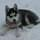It seems that you're using an outdated browser. Some things may not work as they should (or don't work at all).
We suggest you upgrade newer and better browser like: Chrome, Firefox, Internet Explorer or Opera

×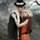GameRager: If you don't want to play and the new guys get spots already i'll take it gladly.
By all means, take it!
stuff changed, i can take part late sundayGameRager: That works too.

Also an aside: I love that game series....nice pic....gonna have to swipe a copy.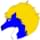RedFireGaming: PS2 or PS3 version? I love it too.
I only have the PS2 originals, though I hear the PS3 versions are good as well. I finished the first game, most of the second, and most of the third(lost track of playing so never finished them fully). Still need to play the more recent ones as well.

Addition: I also have the 3d glasses for the one game.....fun times.

=============GameRager: If you don't want to play and the new guys get spots already i'll take it gladly.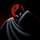dedoporno: By all means, take it!
You sure? If you don't mind and i'm not imposing(and OP is okay with it) that seems ok.
Post edited September 28, 2019 by GameRagerGameRager: If you don't mind and i'm not imposing(and OP is okay with it) that seems ok.
I really don't, take it.GameRager: If you don't mind and i'm not imposing(and OP is okay with it) that seems ok.dedoporno: I really don't, take it.
Thanks once again for being so generous......now if Joe approves and this game starts soon we cna get the proverbial ball rolling.
One more thing. Before we start, I want a room with a view.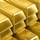ZFR: While we wait:

The unbeatable duo want to determine whom to send to do the NK. Problem is, *someone* doesn't want to use random.org; he loves rolling dice instead. He says that if he rolls an even number then the prettier half of the duo does the NK, and if he rolls an odd number then the smarter half will do it. Problem is, the only dice he has is clearly unbalanced: some numbers are more likely to come than others. Fortunately, the smarter half of the unbeatable duo devices a clever method of using the unbalanced dice to randomly pick one of them with a 50% probability. How?

(This actually has a proper mathematical solution).
Hmmm... no takers?

You roll twice. If the parity is same, discard and roll twice again. Otherwise use the parity of first roll to determine the outcome.

So
even, even - discard, reroll twice
odd, odd - discard, reroll twice
even, odd - ZFR
odd, even - Joe

Since P(even, odd) is equal to P(odd, even), regardless of P(even) and P(odd), both players are picked with an equal 50% chance.

This works for any situation where a dice/coin/roulette_wheel needen't be fair. You can pick any two events, even if their probability is radically disproportional e.g. dice rolls 6 vs dice rolls non-6, just as long as P(x,y) is greater than 0 and both rolls are independent. However the more disproportional they are, the more likely you'll need to discard and reroll.ZFR: While we wait:

The unbeatable duo want to determine whom to send to do the NK. Problem is, *someone* doesn't want to use random.org; he loves rolling dice instead. He says that if he rolls an even number then the prettier half of the duo does the NK, and if he rolls an odd number then the smarter half will do it. Problem is, the only dice he has is clearly unbalanced: some numbers are more likely to come than others. Fortunately, the smarter half of the unbeatable duo devices a clever method of using the unbalanced dice to randomly pick one of them with a 50% probability. How?

(This actually has a proper mathematical solution).ZFR: This works for any situation where a dice/coin/roulette_wheel needen't be fair. You can pick any two events, even if their probability is radically disproportional e.g. dice rolls 6 vs dice rolls non-6, just as long as P(x,y) is greater than 0 and both rolls are independent. However the more disproportional they are, the more likely you'll need to discard and reroll.
OHO! I get it! I’d got as far as “roll the dice twice” but couldn’t think of the next step.ZFR: This works for any situation where a dice/coin/roulette_wheel needen't be fair. You can pick any two events, even if their probability is radically disproportional e.g. dice rolls 6 vs dice rolls non-6, just as long as P(x,y) is greater than 0 and both rolls are independent. However the more disproportional they are, the more likely you'll need to discard and reroll.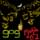JoeSapphire: OHO! I get it! I’d got as far as “roll the dice twice” but couldn’t think of the next step.
Come to think of it, this might work even if the two rolls are not independent. Consider a bag with with a different number of white and black marbles. We take one then another without replacing. Obviously the the probabilities of the second draw are dependent on what ball we pick in the first draw, but P(b,w) is still equal to P(w, b). So the method works nonetheless

P(b,w) = (b/n) * (w/(n-1)) = (bw) / n(n-1)
P(w,b) = (w/n) * (b/ (n-1)) = (bw) / n(n-1)JoeSapphire: OHO! I get it! I’d got as far as “roll the dice twice” but couldn’t think of the next step.ZFR: Come to think of it, this might work even if the two rolls are not independent. Consider a bag with with a different number of white and black marbles. We take one then another without replacing. Obviously the the probabilities of the second draw are dependent on what ball we pick in the first draw, but P(b,w) is still equal to P(w, b). So the method works nonetheless

P(b,w) = (b/n) * (w/(n-1)) = (bw) / n(n-1)
P(w,b) = (w/n) * (b/ (n-1)) = (bw) / n(n-1)
That bit about colored marbles reminds me of that bit in storm of the century(or similar titled film) where the bad dude has the people pick lots with all white marbles except one black one.ZFR: Now for the proof. If you google (or visit your library) you can find quite a few of them. Here is my favourite that uses recursion.
There is an event with a probability p. We need to find on average how many times we need to roll to get p.

Assume that the average is x.

Now, there is a probability p of getting it on first try. And a probability of not getting it on first try is (1-p).
If we don't get it on the first try, then we used up one try but, since x is the answer, then on average it takes x further tries to get it.

Taking that into account, we got a simple equation to solve.
x = (p * 1) + ((1-p) * (1+x))

Which we can solve to get
x = 1/p
I just realized something. Although the above is correct, technically it's not complete. I've proven that if such an average exists, then it's equal to 1/p but I haven't proven that such an average actually does exist.

In Joe's case, such an average does exist because P(infinity) = 0 (the probability that Joe will keep rolling forever is equal to 0). Proof? That's for you to find out.

However consider this game: You roll a d6 dice. If you roll 1, you win. If not, you roll again, only this time the number of dice faces is doubled. And so on. You roll d12 a second time, d24 the third time, d48 the fourth time... etc. You keep rolling until you roll 1.
In this case P(infinity) > 0. For bonus points prove this and calculate P(infinity); it's actually not that difficult.
And because of that, the average number of rolls doesn't exist. Or you could say the average number of rolls is "infinite", but that's not strictly true, because there is no number called "infinity", so the average can't be equal to that.

Anyway, I'll stop now.